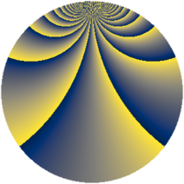# Properties

 Label 2880.2.cuLevel $2880$ Weight $2$ Character orbit 2880.cu Rep. character $\chi_{2880}(49,\cdot)$ Character field $\Q(\zeta_{12})$ Dimension $560$ Sturm bound $1152$

# Learn more about

## Defining parameters

 Level: $$N$$ $$=$$ $$2880 = 2^{6} \cdot 3^{2} \cdot 5$$ Weight: $$k$$ $$=$$ $$2$$ Character orbit: $$[\chi]$$ $$=$$ 2880.cu (of order $$12$$ and degree $$4$$) Character conductor: $$\operatorname{cond}(\chi)$$ $$=$$ $$720$$ Character field: $$\Q(\zeta_{12})$$ Sturm bound: $$1152$$

## Dimensions

The following table gives the dimensions of various subspaces of $$M_{2}(2880, [\chi])$$.

Total New Old
Modular forms 2368 592 1776
Cusp forms 2240 560 1680
Eisenstein series 128 32 96

## Trace form

 $$560q - 2q^{5} + O(q^{10})$$ $$560q - 2q^{5} + 4q^{11} + 8q^{15} + 16q^{19} + 4q^{21} - 4q^{29} + 8q^{31} - 12q^{35} - 14q^{45} - 240q^{49} - 16q^{51} + 4q^{59} - 4q^{61} - 4q^{65} + 4q^{69} - 58q^{75} + 8q^{79} - 16q^{81} + 8q^{85} - 40q^{91} + 4q^{95} + 76q^{99} + O(q^{100})$$

## Decomposition of $$S_{2}^{\mathrm{new}}(2880, [\chi])$$ into newform subspaces

The newforms in this space have not yet been added to the LMFDB.

## Decomposition of $$S_{2}^{\mathrm{old}}(2880, [\chi])$$ into lower level spaces

$$S_{2}^{\mathrm{old}}(2880, [\chi]) \cong$$ $$S_{2}^{\mathrm{new}}(720, [\chi])$$$$^{\oplus 3}$$